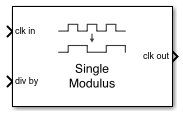Documentation

# Single Modulus Prescaler

Integer clock divider that divides frequency of input signal

• Library:
• Mixed-Signal Blockset / PLL / Building Blocks## Description

The Single Modulus Prescaler subsystem block divides the frequency of the input signal by a tunable integer value, N, passed to the div-by port. In frequency synthesizer circuits, such as a phase-locked loop (PLL) system, these prescalers divide the VCO output frequency by an integer value. The resulting lower frequency at the prescaler output port is comparable to the reference input at the PFD block. The Single Modulus Prescaler is also termed as integer clock divider.

## Ports

### Input

expand all

Input clock frequency, specified as a scalar. In a PLL system, the clk in port is connected to the output port of a VCO block.

Data Types: `double`

Ratio of output to input clock frequency, expressed as a scalar integer.

Data Types: `double`

### Output

expand all

Output clock frequency, expressed as a scalar. In a PLL system, the clk out port is connected to the feedback input port of a PFD block. The output at the clk out port is a square pulse train of 1 V amplitude.

Data Types: `double`

## Parameters

expand all

Select to enable increased buffer size during simulation. This increases the buffer size of the Logic Decision inside the Single Modulus Prescaler block. By default, this option is deselected.

Number of samples of the input buffering available during simulation, specified as a positive integer scalar. This sets the buffer size of the Logic Decision inside the Single Modulus Prescaler block.

Selecting different simulation solver or sampling strategies can change the number of input samples needed to produce an accurate output sample. Set the Buffer size to a large enough value so that the input buffer contains all the input samples required.

#### Dependencies

This parameter is only available when Enable increased buffer size option is selected in the Block Parameters dialog box.

#### Programmatic Use

• Use `get_param(gcb,'NBuffer')` to view the current value of Buffer size.

• Use `set_param(gcb,'NBuffer',value)` to set Buffer size to a specific value.